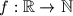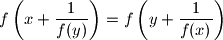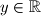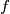### IMO Shortlist 2008 problem A6

Kvaliteta:
Avg: 3,0
Težina:
Avg: 8,0
Let$f: \mathbb{R}\to\mathbb{N}$ be a function which satisfies$f\left(x + \dfrac{1}{f(y)}\right) = f\left(y + \dfrac{1}{f(x)}\right)$ for all$x$,$y\in\mathbb{R}$. Prove that there is a positive integer which is not a value of$f$.

Proposed by Žymantas Darbėnas (Zymantas Darbenas), Lithania
Izvor: Međunarodna matematička olimpijada, shortlist 2008